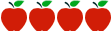### Grade 6: Ratios and Proportional Relationships

Rating:http://schools.nyc.gov/NR/rdonlyres/A9F735CB-47E4-40F8-884F-EA54D0AB5705/0/NYCDOEG6MathRatios_Final.pdf

#### Common Core Standards

Content Standards

6.RP.1 - Understand the concept of a ratio and use ratio language to describe a ratio relationship between two quantities.

6.RP.2 - Understand the concept of a unit rate a/b associated with a ratio a:b with b ≠ 0, and use rate language in the context of a ratio relationship.

6.RP.3 - Use ratio and rate reasoning to solve real-world and mathematical problems, e.g., by reasoning about tables of equivalent ratios, tape diagrams, double number line diagrams, or equations.

6.RP.3a - Make tables of equivalent ratios relating quantities with whole-number measurements, find missing values in the tables, and plot the pairs of values on the coordinate plane. Use tables to compare ratios.

6.RP.3b - Solve unit rate problems including those involving unit pricing and constant speed.

6.RP.3c - Find a percent of a quantity as a rate per 100; solve problems involving finding the whole, given a part and the percent.

6.RP.3d - Use ratio reasoning to convert measurement units; manipulate and transform units appropriately when multiplying or dividing quantities.

Standards for Mathematical Practice

MP.1.     Make sense of problems and persevere in solving them.

MP.2.     Reason abstractly and quantitatively.

MP.3.     Construct viable arguments and critique the reasoning of others.

MP.6.     Attend to precision.

#### Description of Unit

The unit “Ratios and Proportional Relationships” from the NYC Department of Education highlights one of the major focuses for Grade 6.  The sequence of tasks provides students with opportunities to demonstrate and effectively communicate their mathematical understanding of ratios and proportional relationships. Upon completion, students will be able to understand ratio concepts and use ratio reasoning to solve problems.

#### Cautions

Connecticut teachers will need to augment the unit with additional activities. Activities for specific skill development and enrichment are not included. Teachers should also consider making connections to the expressions and equations domain.  The unit makes minimal use of technology and does not include a pre-assessment or self-assessment.

#### Rationale for Selection

The unit is detailed, featuring a unit overview with essential questions and enduring understandings. Most of the instructional materials necessary to teach each lesson are provided. The unit contains explicit details on suggested instructional approaches and overviews, including information on working with students with disabilities and English Language Learners. A performance assessment, grading rubrics, and sample student work are also provided.